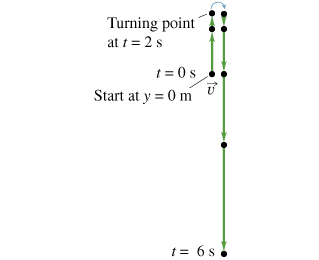# Problem: A stone is thrown upward from the edge of a cliff, reaches its maximum height, and then falls down into the valley below. A motion diagram for this situation is given (Figure 1) , beginning the instant the stone leaves the thrower’s hand.Construct a graph corresponding to the stone's vertical acceleration, ay(t), taking the acceleration due to gravity as exactly 10 m/s2. Ignore air resistance. The unit of time is in seconds and the unit of displacement is in meters. In plotting the points, round-off the coordinate values to the nearest integer.

###### FREE Expert Solution

Acceleration is constant:

g = 10 m/s

At t = 0 s, g = 10 m/s2

At t = 2 s, g = 10 m/s2

At t = 4 s, g = 10 m/s2

At t = 6s, g = 10 m/s2

95% (494 ratings)###### Problem Details

A stone is thrown upward from the edge of a cliff, reaches its maximum height, and then falls down into the valley below. A motion diagram for this situation is given (Figure 1) , beginning the instant the stone leaves the thrower’s hand.

Construct a graph corresponding to the stone's vertical acceleration, ay(t), taking the acceleration due to gravity as exactly 10 m/s2. Ignore air resistance. The unit of time is in seconds and the unit of displacement is in meters. In plotting the points, round-off the coordinate values to the nearest integer.Frequently Asked Questions

What scientific concept do you need to know in order to solve this problem?

Our tutors have indicated that to solve this problem you will need to apply the Vertical Motion and Free Fall concept. You can view video lessons to learn Vertical Motion and Free Fall. Or if you need more Vertical Motion and Free Fall practice, you can also practice Vertical Motion and Free Fall practice problems.